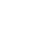注销
• 在线咨询
• 专属顾问
扫码添加产品顾问
为您提供专属答疑• 微信扫码一键拨打
咨询热线： 4008-830-830•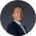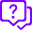0/200

请选择您想要咨询的产品抱歉，您所使用的账号暂未绑定对应的产品！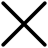• 成为合作伙伴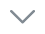• 查询合作伙伴• 合作推广• 了解金蝶• 投资者关系• 新闻与活动• 联系我们请选择您想要咨询的产品计算

整数控件计算有误

int1,int2,int3

int1=1,int2=2,结果int3=0

int1=3,int2=2,结果int3=2

int1=5,int2=2,结果int3=2

int1=7,int2=2,结果int3=4

......

表达式计算出错: FDUN = F_BIS_Decimal /1000

unsupported operand type(s) for //: 'LazyCollection[object, object]' and 'int'

服务:[吨]

（都是明细信息的字段

<

整数控件计算有误

int1,int2,int3

int1=1,int2=2,结果int3=0

int1=3,int2=2,结果int3=2

int1=5,int2=2,结果int3=2

int1=7,int2=2,结果int3=4

......

表达式计算出错: FDUN = F_BIS_Decimal /1000

unsupported operand type(s) for //: 'LazyCollection[object, object]' and 'int'

服务:[吨]

（都是明细信息的字段